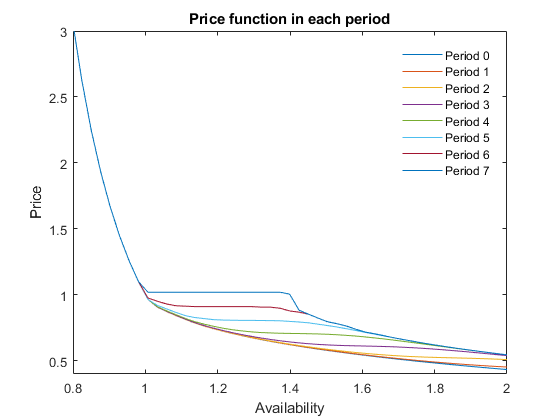# STO3 Anticipated switch to a public storage policy

We consider here the transitional dynamics that occurs when a public storage policy defending a floor price is announced a few periods before its beginning. This is a finite-horizon problem in which the terminal condition is not a unique situation, but a state-contingent policy function. This is a transition from problem STO1 to STO2.

## Create the model objects

Mu                = 1;
sigma             = 0.05;


Competitive storage model:

model1 = recsmodel('sto1.yaml',struct('Mu',Mu,'Sigma',sigma^2,'order',7));

Deterministic steady state (equal to first guess)
State variables:
A
_

1

Response variables:
S    H    P
_    _    _

0    1    1

Expectations variables:
EP    EPe
__    ___

1     1



Floor price model:

model2 = recsmodel('sto2.yaml',struct('Mu',Mu,'Sigma',sigma^2,'order',7));

Deterministic steady state (different from first guess, max(|delta|)=0.392101)
State variables:
A
______

1.3921

Response variables:
S           H       P        Sg
__________    _____    ____    _______

6.0863e-15    1.004    1.02    0.39605

Expectations variables:
EP     EPe
____    ____

1.02    1.02



## Define approximation space

[interp,s] = recsinterpinit(50,min(model1.shocks.e)*0.95,2);


## Terminal condition: solve for the infinite-horizon behavior under policy

See STO2 for details.

Find a first guess through the perfect foresight solution

interp2 = recsFirstGuess(interp,model2,s,[],[],struct('T',5));


Solve for rational expectations

[~,x2] = recsSolveREE(interp2,model2,s);

Successive approximation
Major	 Minor	Lipschitz	 Residual
0	     0	         	 3.69E-02 (Input point)
1	     1	  1.0414	 1.57E-02
2	     1	  0.9613	 9.43E-03
3	     1	  1.0446	 1.89E-03
4	     1	  0.9479	 1.06E-03
5	     1	  0.7874	 2.30E-04
6	     1	  0.8447	 3.57E-05
7	     1	  0.8511	 5.32E-06
8	     1	  0.8498	 7.99E-07
9	     1	  0.8489	 1.21E-07
10	     1	  0.8483	 1.84E-08
11	     1	  1.0000	 0.00E+00
Solution found - Residual lower than absolute tolerance


## Transition: model without policy in finite horizon

The policy is announced 6 periods before it begins (so $T=7$). In the seventh period, the equilibrium is the one defined by the problem with public storage:

T          = 7;
xT         = x2(:,1:3);
options.eqsolver = 'ncpsolve'; % lmmcp does not work in this case
[~,X]  = recsSolveREEFiniteHorizon(interp,model1,s,x2(:,1:3),xT,T,options);


## Initial period: model without policy under infinite horizon

In period 0, the policy is not expected by the agents and the prevailing equilibrium is the one corresponding to the infinite-horizon competitive storage problem (STO1):

[~,x1] = recsSolveREE(interp,model1,s,X(:,1:3,1));

Successive approximation
Major	 Minor	Lipschitz	 Residual
0	     0	         	 3.64E-02 (Input point)
1	     1	  0.8292	 6.25E-03
2	     1	  0.7825	 1.36E-03
3	     1	  0.7646	 3.20E-04
4	     1	  0.7589	 7.72E-05
5	     1	  0.7572	 1.87E-05
6	     1	  0.7567	 4.56E-06
7	     1	  0.7565	 1.11E-06
8	     1	  0.7565	 2.70E-07
9	     1	  0.7565	 6.58E-08
10	     1	  0.7565	 1.60E-08
11	     1	  1.0000	 0.00E+00
Solution found - Residual lower than absolute tolerance


## Plot price function

figure
plot(s,[x1(:,3) squeeze(X(:,3,:))])
xlim([0.8 2])
ylim([0.4 3])
legend([repmat('Period ',T+1,1),num2str((0:T)')])
legend('Location','NorthEast')
legend('boxoff')
xlabel('Availability')
ylabel('Price')
title('Price function in each period')